International
Tables for
Crystallography
Volume B
Reciprocal space
Edited by U. Shmueli

International Tables for Crystallography (2006). Vol. B. ch. 1.2, pp. 22-23   | 1 | 2 |

## Section 1.2.12.2. The cumulant expansion

P. Coppensa*

aDepartment of Chemistry, Natural Sciences & Mathematics Complex, State University of New York at Buffalo, Buffalo, New York 14260-3000, USA
Correspondence e-mail: coppens@acsu.buffalo.edu

#### 1.2.12.2. The cumulant expansion

| top | pdf |

A second statistical expansion which has been used to describe the atomic probability distribution is that of Edgeworth (Kendall & Stuart, 1958; Johnson, 1969). It expresses the function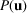as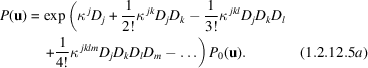Like the moments μ of a distribution, the cumulants κ are descriptive constants. They are related to each other (in the one-dimensional case) by the identity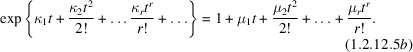When it is substituted for t, (1.2.12.5b)is the characteristic function, or Fourier transform of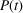(Kendall & Stuart, 1958).

The first two terms in the exponent of (1.2.12.5a)can be omitted if the expansion is around the equilibrium position and the harmonic term is properly described by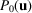.

The Fourier transform of (1.2.12.5a)is, by analogy with the left-hand part of (1.2.12.5b)(with t replaced by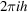),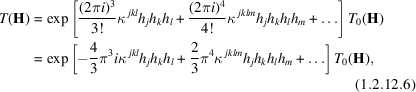where the first two terms have been omitted. Expression (1.2.12.6)is similar to (1.2.12.4)except that the entire series is in the exponent. Following Schwarzenbach (1986), (1.2.12.6)can be developed in a Taylor series, which gives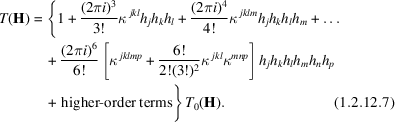This formulation, which is sometimes called the Edgeworth approximation (Zucker & Schulz, 1982), clearly shows the relation to the Gram–Charlier expansion (1.2.12.4), and corresponds to the probability distribution [analogous to (1.2.12.3)]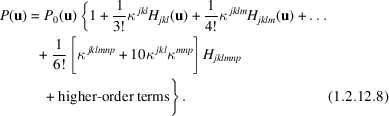The relation between the cumulants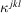and the quasimoments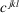are apparent from comparison of (1.2.12.8)and (1.2.12.4):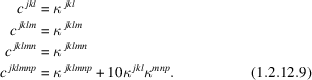The sixth- and higher-order cumulants and quasimoments differ. Thus the third-order cumulantcontributes not only to the coefficient of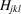, but also to higher-order terms of the probability distribution function. This is also the case for cumulants of higher orders. It implies that for a finite truncation of (1.2.12.6), the probability distribution cannot be represented by a finite number of terms. This is a serious difficulty when a probability distribution is to be derived from an experimental temperature factor of the cumulant type.

### ReferencesJohnson, C. K. (1969). Addition of higher cumulants to the crystallographic structure-factor equation: a generalized treatment for thermal-motion effects. Acta Cryst. A25, 187–194.Google ScholarKendall, M. G. & Stuart, A. (1958). The advanced theory of statistics. London: Griffin.Google ScholarSchwarzenbach, D. (1986). Private communication.Google ScholarZucker, U. H. & Schulz, H. (1982). Statistical approaches for the treatment of anharmonic motion in crystals. I. A comparison of the most frequently used formalisms of anharmonic thermal vibrations. Acta Cryst. A38, 563–568.Google Scholar